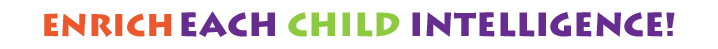Welcome to Our nursery!

•# Explore Our EventsRamadan Activities at IQ Kids Nursery 8:00 AM - 14:00 PM IQ Kids Nursery -Al Shamka Branch, Baniyas Branch & Al Bahya Branch function makeTimer13() { var e = new Date("04/04/2022 8:00:00 AM"), e = Date.parse(e) / 1e3, a = new Date, a = Date.parse(a) / 1e3, t = e - a, o = Math.floor(t / 86400), i = Math.floor((t - 86400 * o) / 3600), s = Math.floor((t - 86400 * o - 3600 * i) / 60), n = Math.floor(t - 86400 * o - 3600 * i - 60 * s); i < "10" && (i = "0" + i), s < "10" && (s = "0" + s), n < "10" && (n = "0" + n), \$("#days13").html(o + "
"), \$("#hours13").html(i + "
"), \$("#minutes13").html(s + "
"), \$("#seconds13").html(n + "
") } setInterval(function() { makeTimer13() }, 1e3); Days Hours Minutes SecondsSummer Term at IQ Kids Nursery Join us for a fun-filled events this Summer at IQ Kids Nursery. function makeTimer8() { var e = new Date("07/05/2021"), e = Date.parse(e) / 1e3, a = new Date, a = Date.parse(a) / 1e3, t = e - a, o = Math.floor(t / 86400), i = Math.floor((t - 86400 * o) / 3600), s = Math.floor((t - 86400 * o - 3600 * i) / 60), n = Math.floor(t - 86400 * o - 3600 * i - 60 * s); i < "10" && (i = "0" + i), s < "10" && (s = "0" + s), n < "10" && (n = "0" + n), \$("#days8").html(o + "
"), \$("#hours8").html(i + "
"), \$("#minutes8").html(s + "
"), \$("#seconds8").html(n + "
") } setInterval(function() { makeTimer8() }, 1e3); Days Hours Minutes SecondsInternational Day of Happiness 9 AM 1 PM function makeTimer6() { var e = new Date("3/18/2021"), e = Date.parse(e) / 1e3, a = new Date, a = Date.parse(a) / 1e3, t = e - a, o = Math.floor(t / 86400), i = Math.floor((t - 86400 * o) / 3600), s = Math.floor((t - 86400 * o - 3600 * i) / 60), n = Math.floor(t - 86400 * o - 3600 * i - 60 * s); i < "10" && (i = "0" + i), s < "10" && (s = "0" + s), n < "10" && (n = "0" + n), \$("#days6").html(o + "
"), \$("#hours6").html(i + "
"), \$("#minutes6").html(s + "
"), \$("#seconds6").html(n + "
") } setInterval(function() { makeTimer6() }, 1e3); Days Hours Minutes SecondsIQ KIDS NURSERY SPORTSDAY 9 AM 1 PM It's time to get your game on little buddies and be ready for our fun-filled mini event. function makeTimer5() { var e = new Date("2/25/2021"), e = Date.parse(e) / 1e3, a = new Date, a = Date.parse(a) / 1e3, t = e - a, o = Math.floor(t / 86400), i = Math.floor((t - 86400 * o) / 3600), s = Math.floor((t - 86400 * o - 3600 * i) / 60), n = Math.floor(t - 86400 * o - 3600 * i - 60 * s); i < "10" && (i = "0" + i), s < "10" && (s = "0" + s), n < "10" && (n = "0" + n), \$("#days5").html(o + "
"), \$("#hours5").html(i + "
"), \$("#minutes5").html(s + "
"), \$("#seconds5").html(n + "
") } setInterval(function() { makeTimer5() }, 1e3); Days Hours Minutes Seconds